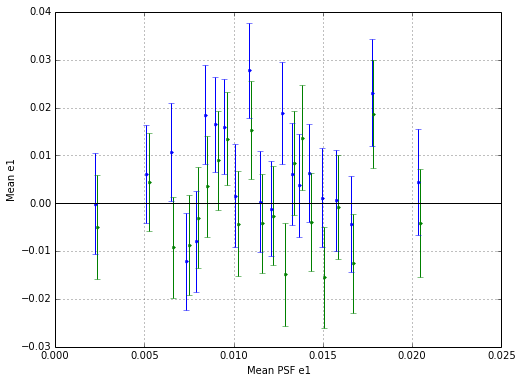# Effect of new astrometry on one tile¶

On 25 July 2014 Erin Sheldon sent this messsage to des-wl-test:

Eli Rykoff has been re-solving the astronomy on some of the coadd tiles and seeing huge improvements. It would be interesting to see if we detect a difference in our shapes.

Over the weekend I ran im3shape on the two tiles he generated. These are the results of comparing the im3shape v6 catalog version of that tile with the new astronomy one.

For reasons I have not yet looked into one of the tiles did not have many objects in it in the re-run version compared to the old one, so this comparison looks at the other tile, DES0453-4748.

A significant issue noted in our shape catalogs is a mean e_1 value > 0 which persists with high S/N. At least on this one tile, that effect appears to go away with the new astrometry.

Cell 6 is the money cell.

### Import dependencies and load and sort tables¶

In :
import astropy.table
import scipy.stats
tile='DES0453-4748'
old_table.sort("identifier")
new_table.sort("identifier")


### Find objects in both old and new cats and cut down both to this set¶

There's some objects that are not in both arrays. I'm not entirely sure why this is - the process I used was the same. Possibly some failures do not occur in one analysis or the other

In :
in_both = set(old_table['identifier']).intersection(new_table['identifier'])
in_old = np.array([x in in_both for x in old_table['identifier']])
in_new = np.array([x in in_both for x in new_table['identifier']])
old_table=old_table[in_old]
new_table=new_table[in_new]


### Find objects which have all flags == 0 in both catalogs¶

The same story - the flags are somewhat different. This is partly due to a problem doing the post-processing on the new astrometry files - I could not extract the coadd objects IDs for them, presumably because the metadata is different from what's found in the nornal files. I haven't investigated thie s properly yet, though.

In :
okay=(old_table['info_flag']==0)&(old_table['error_flag']==0)&(new_table['info_flag']==0)&(new_table['error_flag']==0)
old_table=old_table[okay]
new_table=new_table[okay]


### Plot old e1 versus new e1¶

The black line is y=x. The red line is a least-squares fit (not entirely appropriate since the errors on the shapes are not consistent across e, but not bad) of new to old.

There's a shift of about 1% in the slope, which is important enough, but there is also a 6e-3 shift in the mean value (see below).

In :
figure(figsize=(8,8))
plot(old_table['e1'], new_table['e1'], ',')
x=linspace(-0.8, 0.8, 2)
fit=scipy.stats.linregress(old_table['e1'], new_table['e1'])
slope = fit
intercept = fit
plot(x, x, 'k-')
plot(x, slope*x+intercept, 'r-')
xlim(-0.8, 0.8)
ylim(-0.8, 0.8)
grid()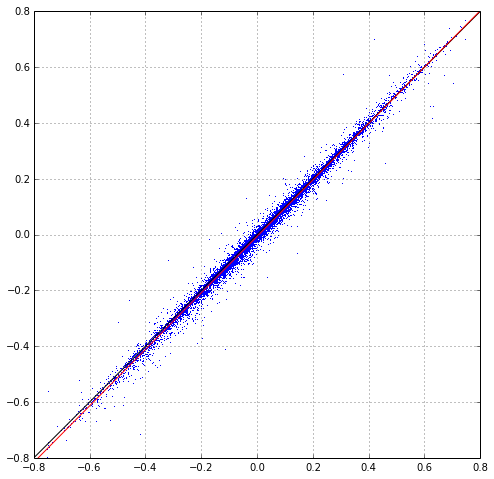### Linear fit of old e1 to new e1¶

In :
print "slope-1, intercept = ", slope-1, intercept

slope-1, intercept =  0.0116507314932 -0.00666677161393


### Mean e1 and e2 in old and new catalogs - MAIN RESULT OF THIS NOTEBOOK!¶

A few summary statistics - this is the fun bit!

In :
print "Old mean e_1 = (%.3f ± %.3f) * 10^-3" % (old_table['e1'].mean()*1e3, old_table['e1'].std()/sqrt(len(old_table))*1e3)
print "New mean e_1 = (%.3f ± %.3f) * 10^-3" % (new_table['e1'].mean()*1e3, new_table['e1'].std()/sqrt(len(new_table))*1e3)

print "Old mean e_2 = (%.3f ± %.3f) * 10^-3" % (old_table['e2'].mean()*1e3, old_table['e2'].std()/sqrt(len(old_table))*1e3)
print "New mean e_2 = (%.3f ± %.3f) * 10^-3" % (new_table['e2'].mean()*1e3, new_table['e2'].std()/sqrt(len(new_table))*1e3)
de1 = new_table['e1'] - old_table['e1']
de2 = new_table['e2'] - old_table['e2']
print
print "Change in e_1 = (%.3f ± %.3f) * 10^-3" % ( de1.mean()*1e3, de1.std()/sqrt(len(old_table))*1e3)
print "Change in e_2 = (%.3f ± %.3f) * 10^-3" % ( de2.mean()*1e3, de2.std()/sqrt(len(old_table))*1e3)

Old mean e_1 = (6.502 ± 2.277) * 10^-3
New mean e_1 = (-0.089 ± 2.313) * 10^-3
Old mean e_2 = (-2.851 ± 2.303) * 10^-3
New mean e_2 = (-1.562 ± 2.340) * 10^-3

Change in e_1 = (-6.591 ± 0.211) * 10^-3
Change in e_2 = (1.289 ± 0.206) * 10^-3


### Histogram of old and new, and plot of the different between them.¶

In :
_=hist(old_table['e1'], bins=50, histtype='step', label='old')
_=hist(new_table['e1'], bins=50, histtype='step', label='new')
legend()
figure(figsize=(8,8))
plot(old_table['e1'], new_table['e1']-old_table['e1'],',')
xlabel("Old e1")
ylabel("Difference new e1 - old e1")
grid()
_=axis('equal')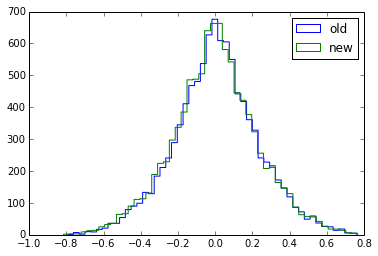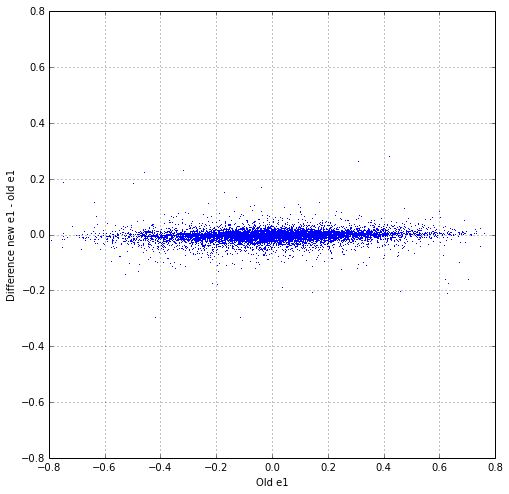### The mean e1 effect was constant with S/N. Here we plot mean e1 in log10(S/N) bins¶

This function is a handy one for binning one quantity in another with equal counts per bin

In :
def mean_in_bins(x, y, n):
xs=np.sort(x)
N=len(xs)
dn=N*1.0/n
dx=xs[-1]-xs
bounds = [xs-dx*0.0001]
for i in xrange(n-1):
si=int((i+1)*dn)
bounds.append(xs[si])
bounds.append(xs[-1]+dx*0.0001)
b=np.digitize(x, bounds)
Y = []
X = []
sY = []
for i in xrange(n):
yi = y[b==i+1]
ni = len(yi)
Y.append(yi.mean())
sY.append(yi.std()/sqrt(ni))
X.append(x[b==i+1].mean())
return np.array(X), np.array(Y), np.array(sY)


### First with 21 bins in SNR¶

Anyone have any idea what's happening between log10(snr)=1.8 and log10(snr)=2.0 (snr in 60 - 100)? I plotted these objects in RA and Dec and there was no obvious group of objects (e.g. from a sattelite/plane trail)

In :
figure(figsize=(8,6))
X, Y, sY = mean_in_bins(log10(old_table['snr']), old_table['e1'], 21)
_=errorbar(X-0.005, Y, sY, fmt='.', label="Old")
X, Y, sY = mean_in_bins(log10(new_table['snr']), new_table['e1'], 21)
_=errorbar(X+0.005, Y, sY, fmt='.', label="New")
xl=xlim()
plot(xl, [0,0],'k-')
grid()
xlabel("Log10(SNR)")
ylabel("Mean e1")
_=xlim(*xl)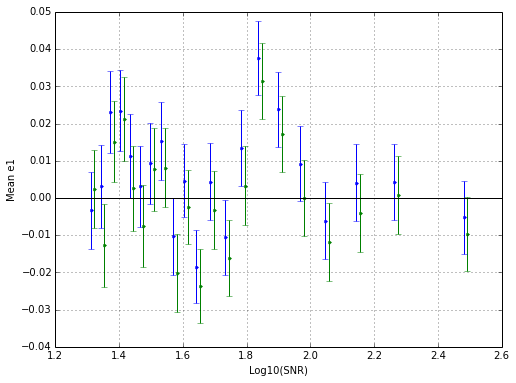### And now with just 4 bins:¶

In :
figure(figsize=(8,6))
X, Y, sY = mean_in_bins(log10(old_table['snr']), old_table['e1'], 4)
_=errorbar(X-0.005, Y, sY, fmt='.', label="Old")
X, Y, sY = mean_in_bins(log10(new_table['snr']), new_table['e1'], 4)
_=errorbar(X+0.005, Y, sY, fmt='.', label="New")
xl=xlim()
plot(xl, [0,0],'k-')
grid()
xlabel("Log10(SNR)")
ylabel("Mean e1")
_=xlim(*xl)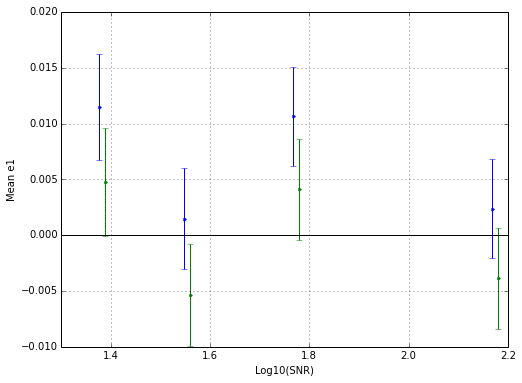### And just to check for any visible effect with PSF e1, though there's not really enough data here to see:¶

In :
figure(figsize=(8,6))
X, Y, sY = mean_in_bins(old_table['mean_psf_e1_sky'], old_table['e1'], 21)
_=errorbar(X-0.00005, Y, sY, fmt='.', label="Old")
X, Y, sY = mean_in_bins(new_table['mean_psf_e1_sky'], new_table['e1'], 21)
_=errorbar(X+0.00005, Y, sY, fmt='.', label="New")
xl=xlim()
plot(xl, [0,0],'k-')
grid()
xlabel("Mean PSF e1")
ylabel("Mean e1")
_=xlim(*xl)